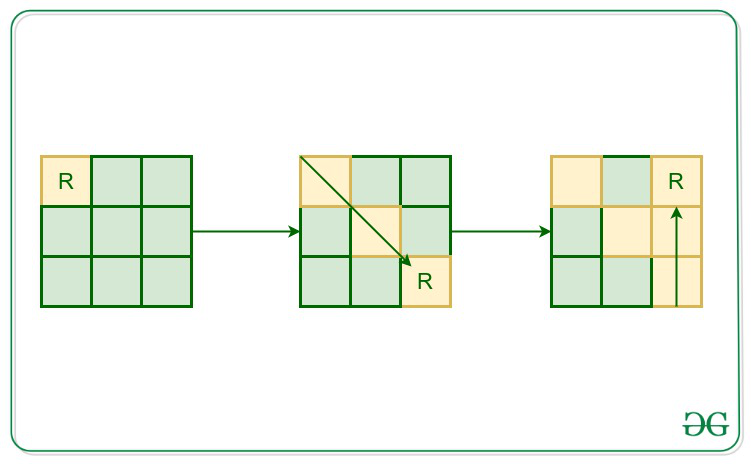Related Articles
Find cells in Matrix that are not visited by Robot for given movements
• Last Updated : 27 Oct, 2020

Given an integer N, which denotes the size of the matrix that is N*N. There is a robot placed over the top-left corner (0, 0) of the matrix, the direction of movement of the robot are given as (N, S, W, E, NE, NW, SE, SW which denotes North, South, West, East, North-East, North-west, South-east, South-west respectively) and the duration of the movement in a particular direction is also given. The task is to find the unvisited cells of the matrix after the movement of the robot is completed at the end of all winds.

Note: Robot can visit a cell only once. If at any point robot cannot move then it will stay at its current position. Also, robot can make one move per second.

Examples:

Input: N = 3, move[] = {(0, SE), (2, N)}
Output: 4
Explanation:Input:
N = 5,  move[] =
{(0, SE),
(1, NE),
(2, E),
(6, SW),
(15, N),
(20, W)}
Output:
13
Explanation:
After the movements of the robot, there are 13 Cells unvisited.

Approach: The idea is to use recursion to solve this problem. Initially, set the current position of the robot as the (0, 0). Start the movement of the robot as per the given direction and mark the cells as visited of the matrix. Finally, After the complete movement of the robot mark count the cells of the matrix which are not marked as visited

Below code implements the approach discussed above:

## C++

 `// C++ implementation to find the``// unvisited cells of the matrix` `#include ` `using` `namespace` `std;` `// Dimension``// of the board``int` `n;` `// Current location``// of the robot``int` `curr_i = 0, curr_j = 0;` `// Function to move the robot``void` `moveRobot(``    ``int` `n, ``int` `i,``    ``int` `j, ``int` `dx,``    ``int` `dy, ``int``& duration,``    ``vector >& visited)``{` `    ``// if the robot tends to move``    ``// out of the board``    ``// or tends to visit an``    ``// already visited position``    ``// or the wind direction is changed``    ``if` `(i < 0 || i >= n || j < 0 || j >= n``        ``|| visited[i][j] == ``true``        ``|| duration == 0) {` `        ``// the robot can't move further``        ``// under the influence of``        ``// current wind direction``        ``return``;``    ``}` `    ``// Change the current location``    ``// and mark the current``    ``// position as visited``    ``curr_i = i;``    ``curr_j = j;``    ``visited[i][j] = ``true``;` `    ``// One second passed``    ``// visiting this position``    ``duration--;` `    ``moveRobot(n, i + dx, j + dy, dx,``              ``dy, duration, visited);``}` `// Function to find the unvisited``// cells of the matrix after movement``void` `findUnvisited(``    ``int` `p,``    ``vector > periods)``{``    ``// nXn matrix to store the``    ``// visited state of positions``    ``vector > visited;` `    ``// map to store the wind directions``    ``unordered_map > mp``        ``= { { ``"N"``, { -1, 0 } },``            ``{ ``"S"``, { 1, 0 } },``            ``{ ``"E"``, { 0, 1 } },``            ``{ ``"W"``, { 0, -1 } },``            ``{ ``"NE"``, { -1, 1 } },``            ``{ ``"NW"``, { -1, -1 } },``            ``{ ``"SE"``, { 1, 1 } },``            ``{ ``"SW"``, { 1, -1 } } };` `    ``// Initially all of the``    ``// positions are unvisited``    ``for` `(``int` `i = 0; i < n; i++) {``        ``visited.push_back(vector<``bool``>{});``        ``for` `(``int` `j = 0; j < n; j++) {``            ``visited[i].push_back(``false``);``        ``}``    ``}` `    ``for` `(``int` `i = 0; i < p; i++) {``        ``string dir = periods[i].second;``        ``int` `dx = mp[dir];``        ``int` `dy = mp[dir];` `        ``// duration for the which the``        ``// current direction of wind exists``        ``int` `duration;` `        ``if` `(i < p - 1) {``            ``// difference of the start time``            ``// of current wind direction``            ``// and start time of the``            ``// upcoming wind direction``            ``duration``                ``= periods[i + 1].first``                  ``- periods[i].first;``        ``}``        ``else` `{``            ``// the maximum time for which``            ``// a robot can move is``            ``// equal to the diagonal``            ``// length of the square board``            ``duration = ``sqrt``(2) * n;``        ``}` `        ``// If its possible to move``        ``// the robot once in the``        ``// direction of wind, then``        ``// move it once and call the``        ``// recursive function for``        ``// further movements``        ``int` `next_i = curr_i + dx;``        ``int` `next_j = curr_j + dy;` `        ``if` `(next_i >= 0``            ``&& next_i < n``            ``&& next_j >= 0``            ``&& next_j < n``            ``&& visited[next_i][next_j] == ``false``            ``&& duration > 0) {``            ``moveRobot(n, next_i,``                      ``next_j, dx, dy,``                      ``duration, visited);``        ``}``    ``}` `    ``// Variable to store the``    ``// number of unvisited positions``    ``int` `not_visited = 0;` `    ``// traverse over the matrix and``    ``// keep counting the unvisited positions``    ``for` `(``int` `i = 0; i < n; i++) {``        ``for` `(``int` `j = 0; j < n; j++) {``            ``if` `(visited[i][j] == ``false``) {``                ``not_visited++;``            ``}``        ``}``    ``}` `    ``cout << not_visited << ``"\n"``;``}` `// Driver Code``int` `main()``{` `    ``// Dimension of the board``    ``n = 5;` `    ``// number of periods``    ``int` `p = 6;` `    ``// vector of pairs``    ``vector > periods(p);``    ``periods = { 0, ``"SE"` `};``    ``periods = { 1, ``"NE"` `};``    ``periods = { 2, ``"E"` `};``    ``periods = { 6, ``"SW"` `};``    ``periods = { 15, ``"N"` `};``    ``periods = { 20, ``"W"` `};` `    ``// Function Call``    ``findUnvisited(p, periods);``    ``return` `0;``}`
Output:
```13

```

Attention reader! Don’t stop learning now. Get hold of all the important DSA concepts with the DSA Self Paced Course at a student-friendly price and become industry ready.

My Personal Notes arrow_drop_up